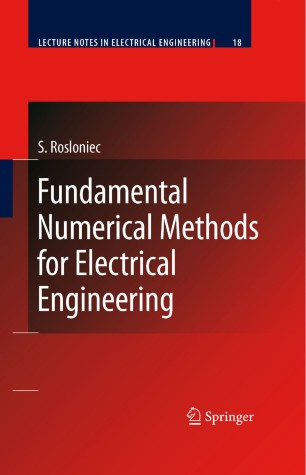# Fundamental Numerical Methods for Electrical Engineering

• Stanisław RosłoniecBook

Part of the Lecture Notes Electrical Engineering book series (LNEE, volume 18)

1. Front Matter
Pages I-XIII
2. Stanisław Rosłoniec
Pages 1-27
3. Stanisław Rosłoniec
Pages 29-48
4. Stanisław Rosłoniec
Pages 49-68
5. Stanisław Rosłoniec
Pages 69-120
6. Stanisław Rosłoniec
Pages 121-154
7. Stanisław Rosłoniec
Pages 155-177
8. Stanisław Rosłoniec
Pages 179-222
9. Stanisław Rosłoniec
Pages 223-268
10. Back Matter
Pages 269-283

### Introduction

The book presents fundamental numerical methods which are most frequently applied in the electrical (electronic) engineering. A scope of this book is rather wide and includes solving the sets of linear and nonlinear equations, interpolation and approximation of the functions of one variable, integration and differentation of the functions of one and two variables, integration of the ordinary differential equations, and integration the partial differential equations of two variables. All methods discussed are illustrated with real-world examples of applications. It is shown how real engineering questions can be transformed into the corresponding mathematical problems and next effectively solved by using appropriate numerical methods. Usually, for teaching reasons, mathematical problems are solved step by step, and illustrated by neumerous intermediate results. Thus, it is not only explained how the problem can be solved, but the details of the solution are also demonstrated. In Chapter 7 for instance three electrical rectifying devices and a transmission-line non- homogeneous impedance transformer are analyzed in this manner. Similarly, in Chapter 8 different aspects of Laplace boundary value problem formulated for various kinds of single and coupled TEM transmission lines are presented. In other words, six standard TEM transmission lines are investigated by means of the finite difference method. It is shown how a partial differential equation of the second order can be approximated by the corresponding difference equation defined on interior and boundary nodes of the introduced grid. At the next stage, the set of linear equations, formulated in this way, is recurrently solved by using the effective SOR technique. Additionally, ideas of " fictitious nodes " and the " even and odd mode excitations " methods are explained and illustrated. All methods and computational results, presented in the book, are of significant practical value. Thus, this book may be especially helpful for students of electronic departments of technical universities and for practicing engineers.

### Keywords

Software Standard automation computer computer-aided design (CAD) computer-aided manufacturing (CAM) development differential equation electrical engineering manufacturing model numerical methods ordinary differential equation production

#### Authors and affiliations

• Stanisław Rosłoniec
• 1
1. 1.Institute of RadioelectronicsWarsaw University of TechnologyNowowiejska 15/19Poland

### Bibliographic information

• DOI https://doi.org/10.1007/978-3-540-79519-3
• Copyright Information Springer-Verlag Berlin Heidelberg 2008
• Publisher Name Springer, Berlin, Heidelberg
• eBook Packages Engineering
• Print ISBN 978-3-540-79518-6
• Online ISBN 978-3-540-79519-3
• Series Print ISSN 1876-1100
• Buy this book on publisher's site